# You measure the angle from the ground to the top of the building from a point 70 feet away from the base of the building. The true value of the angle is 60 degrees, but your measurement may be in error by as much as ±2∘. Assuming level ground and a plumb building, use differentials to approximate the maximum possible error in calculating the height of the building using your measurement of the angle. Round your answer to three decimal places.

Question
21 views

You measure the angle from the ground to the top of the building from a point 70 feet away from the base of the building. The true value of the angle is 60 degrees, but your measurement may be in error by as much as ±2∘. Assuming level ground and a plumb building, use differentials to approximate the maximum possible error in calculating the height of the building using your measurement of the angle. Round your answer to three decimal places.

check_circle

Step 1

Given:

Base, x=70 ft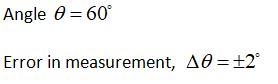Let height of the building = h

A rough sketch of the given problem is as follows: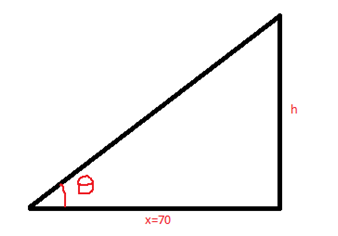Step 2

In the above triangle: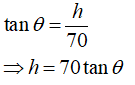Error in h is given as: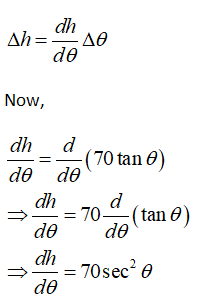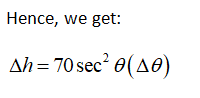Step 3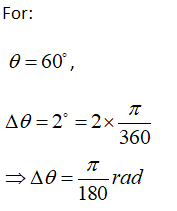We get: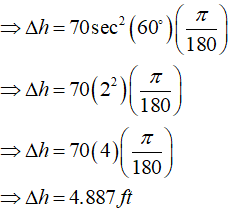...

### Want to see the full answer?

See Solution

#### Want to see this answer and more?

Solutions are written by subject experts who are available 24/7. Questions are typically answered within 1 hour.*

See Solution
*Response times may vary by subject and question.
Tagged in